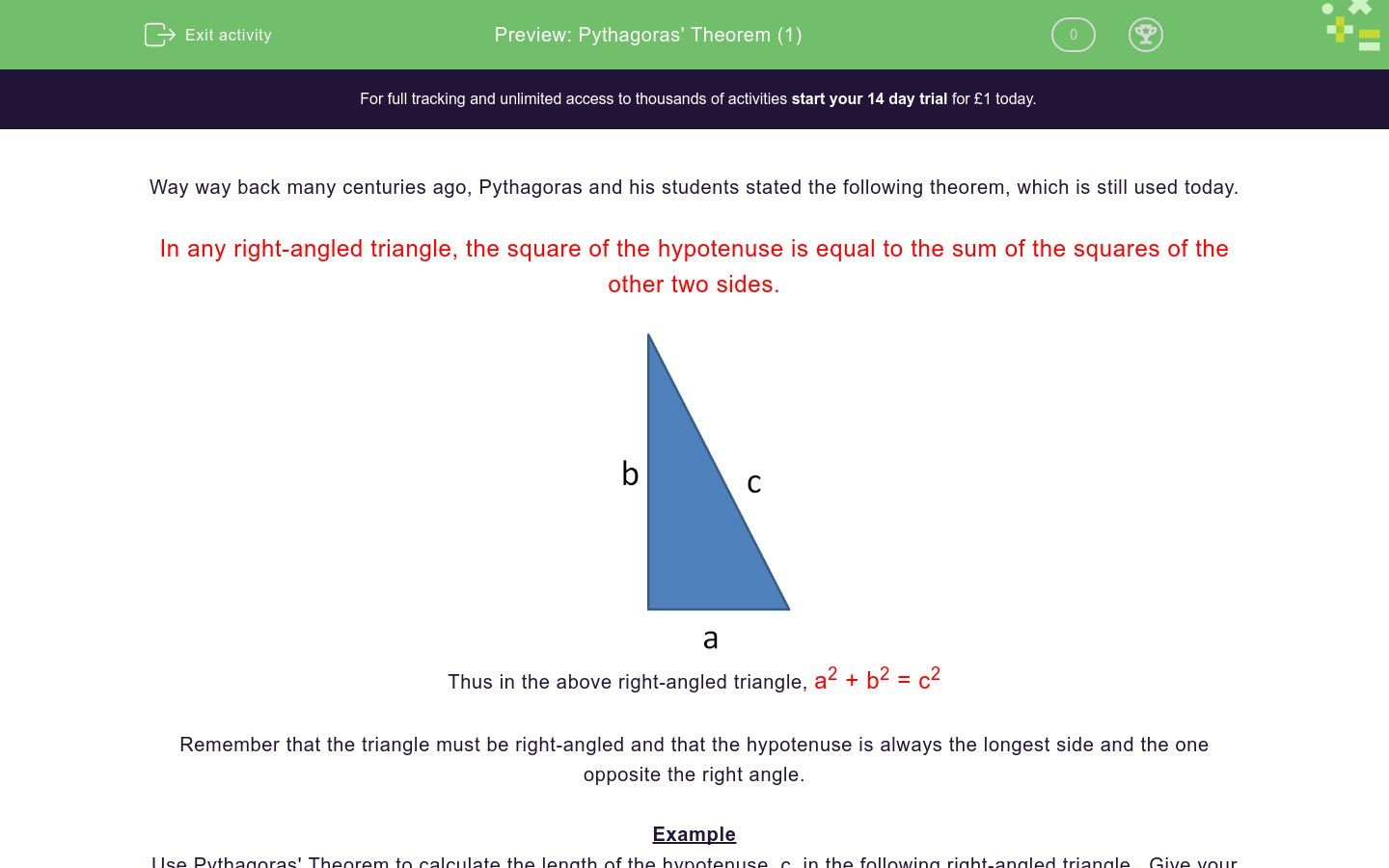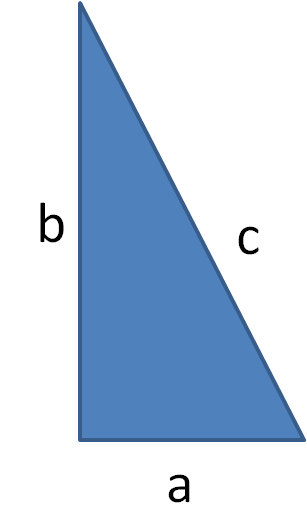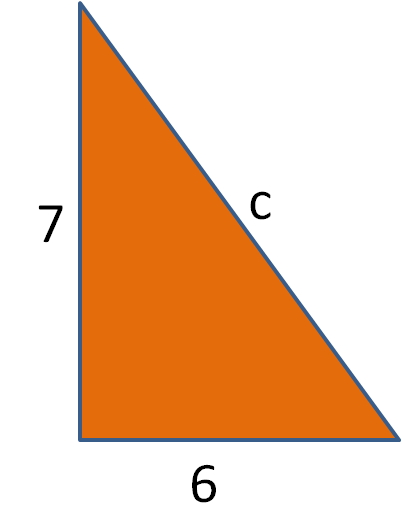# Pythagoras' Theorem (1)

In this worksheet, students find the length of the hypotenuse of right-angled triangles using Pythagoras' Theorem.Key stage:  KS 3

Curriculum topic:   Geometry and Measures

Curriculum subtopic:   Solve Right-Angled Triangle Problems

Difficulty level:### QUESTION 1 of 10

Way way back many centuries ago, Pythagoras and his students stated the following theorem, which is still used today.

In any right-angled triangle, the square of the hypotenuse is equal to the sum of the squares of the other two sides.Thus in the above right-angled triangle, a2 + b2 = c2

Remember that the triangle must be right-angled and that the hypotenuse is always the longest side and the one opposite the right angle.

Example

Use Pythagoras' Theorem to calculate the length of the hypotenuse, c, in the following right-angled triangle.  Give your answer to 3 significant figures.By Pythagoras' Theorem,

c2 = 32 + 82

c2 = 9 + 64

c2 = 73

c = √73

c = 8.54 (to 3 s.f.)

Use Pythagoras' Theorem to calculate the length of the hypotenuse, c, in the following right-angled triangle.  Give your answer to 3 significant figures.Use Pythagoras' Theorem to calculate the length of the hypotenuse, c, in the following right-angled triangle.  Give your answer to 3 significant figures.Use Pythagoras' Theorem to calculate the length of the hypotenuse, c, in the following right-angled triangle.  Give your answer to 3 significant figures.Use Pythagoras' Theorem to calculate the length of the hypotenuse, c, in the following right-angled triangle.  Give your answer to 3 significant figures.Use Pythagoras' Theorem to calculate the length of the hypotenuse, c, in the following right-angled triangle.  Give your answer to 3 significant figures.Use Pythagoras' Theorem to calculate the length of the hypotenuse, c, in the following right-angled triangle.  Give your answer to 3 significant figures.Use Pythagoras' Theorem to calculate the length of the hypotenuse, c, in the following right-angled triangle.  Give your answer to 3 significant figures.Use Pythagoras' Theorem to calculate the length of the hypotenuse, c, in the following right-angled triangle.  Give your answer to 3 significant figures.Use Pythagoras' Theorem to calculate the length of the hypotenuse, c, in the following right-angled triangle.  Give your answer to 3 significant figures.Use Pythagoras' Theorem to calculate the length of the hypotenuse, c, in the following right-angled triangle.  Give your answer to 3 significant figures.• Question 1

Use Pythagoras' Theorem to calculate the length of the hypotenuse, c, in the following right-angled triangle.  Give your answer to 3 significant figures.6.71
EDDIE SAYS

c2 = 32 + 62

c2 = 9 + 36

c2 = 45

c = √45

• Question 2

Use Pythagoras' Theorem to calculate the length of the hypotenuse, c, in the following right-angled triangle.  Give your answer to 3 significant figures.7.21
EDDIE SAYS

c2 = 42 + 62

c2 = 16 + 36

c2 = 52

c = √52

• Question 3

Use Pythagoras' Theorem to calculate the length of the hypotenuse, c, in the following right-angled triangle.  Give your answer to 3 significant figures.8.60
EDDIE SAYS

c2 = 52 + 72

c2 = 25 + 49

c2 = 74

c = √74

• Question 4

Use Pythagoras' Theorem to calculate the length of the hypotenuse, c, in the following right-angled triangle.  Give your answer to 3 significant figures.9.22
EDDIE SAYS

c2 = 62 + 72

c2 = 36 + 49

c2 = 85

c = √85

• Question 5

Use Pythagoras' Theorem to calculate the length of the hypotenuse, c, in the following right-angled triangle.  Give your answer to 3 significant figures.10.8
EDDIE SAYS

c2 = 62 + 92

c2 = 36 + 81

c2 = 117

c = √117

• Question 6

Use Pythagoras' Theorem to calculate the length of the hypotenuse, c, in the following right-angled triangle.  Give your answer to 3 significant figures.12.5
EDDIE SAYS

c2 = 62 + 112

c2 = 36 + 121

c2 = 157

c = √157

• Question 7

Use Pythagoras' Theorem to calculate the length of the hypotenuse, c, in the following right-angled triangle.  Give your answer to 3 significant figures.6.32
EDDIE SAYS

c2 = 22 + 62

c2 = 4 + 36

c2 = 40

c = √40

• Question 8

Use Pythagoras' Theorem to calculate the length of the hypotenuse, c, in the following right-angled triangle.  Give your answer to 3 significant figures.10.4
EDDIE SAYS

c2 = 32 + 102

c2 = 9 + 100

c2 = 109

c = √109

• Question 9

Use Pythagoras' Theorem to calculate the length of the hypotenuse, c, in the following right-angled triangle.  Give your answer to 3 significant figures.11.2
EDDIE SAYS

c2 = 52 + 102

c2 = 25 + 100

c2 = 125

c = √125

• Question 10

Use Pythagoras' Theorem to calculate the length of the hypotenuse, c, in the following right-angled triangle.  Give your answer to 3 significant figures.7.07
EDDIE SAYS

c2 = 52 + 52

c2 = 25 + 25

c2 = 50

c = √50

---- OR ----